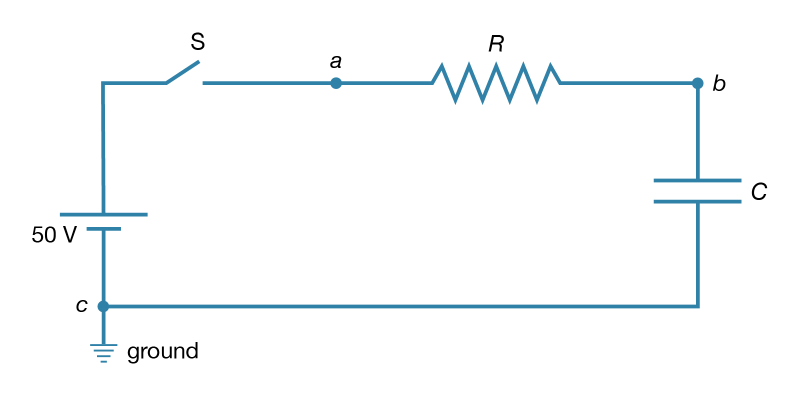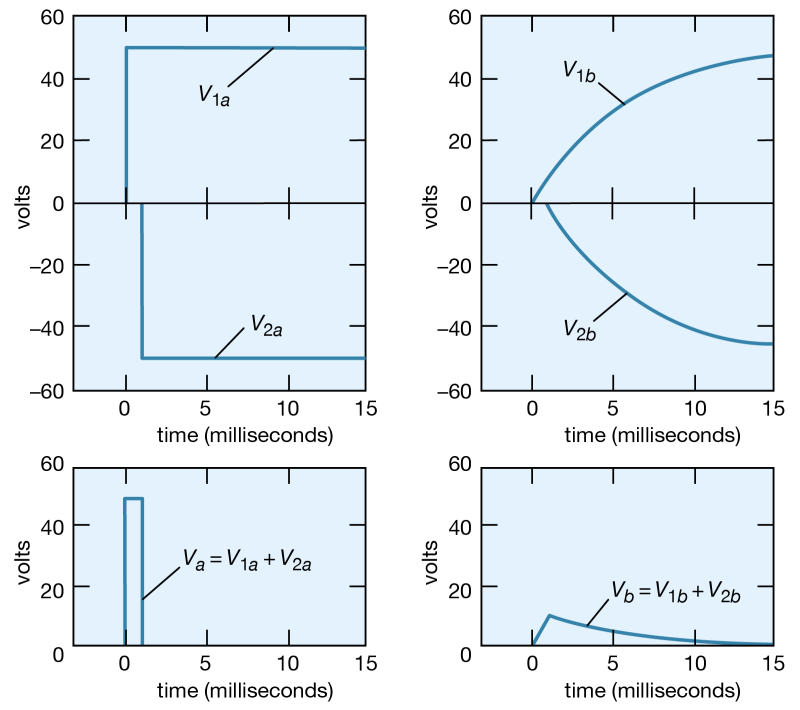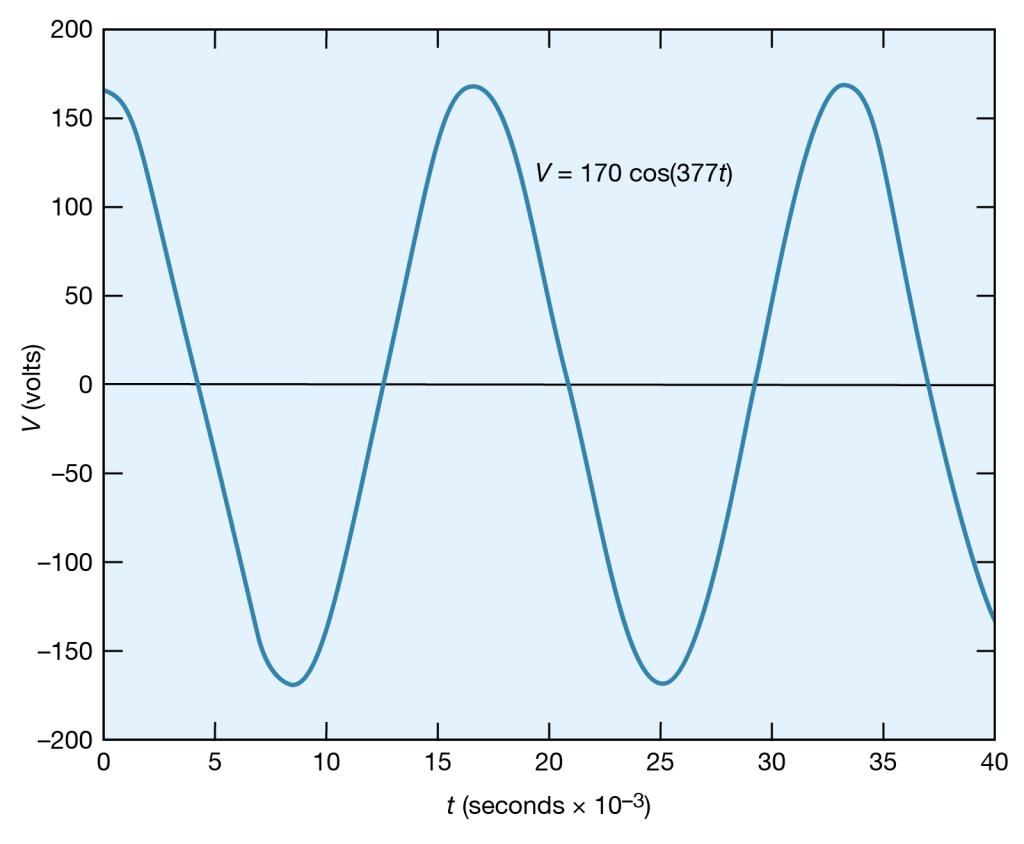# Alternating Electric Currents Physics Notes | EduRev

## Physics : Alternating Electric Currents Physics Notes | EduRev

The document Alternating Electric Currents Physics Notes | EduRev is a part of the Physics Course Basic Physics for IIT JAM.
All you need of Physics at this link: Physics

Introduction:

Alternating current, abbreviation AC, flow of electric charge that periodically reverses. It starts, say, from zero, grows to a maximum, decreases to zero, reverses, reaches a maximum in the opposite direction, returns again to the original value, and repeats this cycle indefinitely. The interval of time between the attainment of a definite value on two successive cycles is called the period, the number of cycles or periods per second is the frequency, and the maximum value in either direction is the amplitude of the alternating current. Low frequencies, such as 50 and 60 cycles per second (hertz), are used for domestic and commercial power, but alternating currents of frequencies around 100,000,000 cycles per second (100 megahertz) are used in television and those of several thousand megahertz in radar or microwave communication. Cellular telephones operate at frequencies of about 1,000 megahertz (1 gigahertz).
Alternating current (AC) has the distinct advantage over direct current (DC; a steady flow of electric charge in one direction) of being able to transmit power over large distances without great loss of energy to resistance. The power transmitted is equal to the current times the voltage; however, the power lost is equal to the resistance times the square of the current. Changing voltages was very difficult with the first DC electric power grids in the late 19th century. Because of the power loss, these grids used low voltages to maintain high current and thus could only transmit usable power over short distances. DC power transmission was soon supplanted by AC systems that transmit power at very high voltages (and correspondingly low current) and easily use transformers to change the voltage. Current systems transmit power from generators at hundreds of thousands of volts and use transformers to lower the voltage to 220 volts (as in much of the world) or 120 volts (as in North America) for individual customers.

Basic Phenomena and Principles:

Many applications of electricity and magnetism involve voltages that vary in time. Electric power transmitted over large distances from generating plants to users involves voltages that vary sinusoidally in time, at a frequency of 60 hertz (Hz) in the United States and Canada and 50 hertz in Europe. (One hertz equals one cycle per second.) This means that in the United States, for example, the current alternates its direction in the electric conducting wires so that each second it flows 60 times in one direction and 60 times in the opposite direction. Alternating currents (AC) are also used in radio and television transmissions. In an AM (amplitude-modulation) radio broadcast, electromagnetic waves with a frequency of around one million hertz are generated by currents of the same frequency flowing back and forth in the antenna of the station. The information transported by these waves is encoded in the rapid variation of the wave amplitude. When voices and music are broadcast, these variations correspond to the mechanical oscillations of the sound and have frequencies from 50 to 5,000 hertz. In an FM (frequency-modulation) system, which is used by both television and FM radio stations, audio information is contained in the rapid fluctuation of the frequency in a narrow range around the frequency of the carrier wave.
Circuits that can generate such oscillating currents are called oscillators; they include, in addition to transistors, such basic electrical components as resistors, capacitors, and inductors. As was mentioned above, resistors dissipate heat while carrying a current. Capacitors store energy in the form of an electric field in the volume between oppositely charged electrodes. Inductors are essentially coils of conducting wire; they store magnetic energy in the form of a magnetic field generated by the current in the coil. All three components provide some impedance to the flow of alternating currents. In the case of capacitors and inductors, the impedance depends on the frequency of the current. With resistors, impedance is independent of frequency and is simply the resistance. This is easily seen from Ohm’s law, equation (21), when it is written as i = V/R. For a given voltage difference V between the ends of a resistor, the current varies inversely with the value of R. The greater the value R, the greater is the impedance to the flow of electric current. Before proceeding to circuits with resistors, capacitors, inductors, and sinusoidally varying electromotive forces, the behaviour of a circuit with a resistor and a capacitor will be discussed to clarify transient behaviour and the impedance properties of the capacitor.

V= iR (21)

Transient Response:

Consider a circuit consisting of a capacitor and a resistor that are connected as shown in Figure 19. What will be the voltage at point b if the voltage at a is increased suddenly from Va = 0 to Va = +50 volts? Closing the switch produces such a voltage because it connects the positive terminal of a 50-volt battery to point a while the negative terminal is at ground (point c). Figure 20 graphs this voltage Va as a function of the time.Figure 19: An RC circuit. This type of electric circuit consists of both a resistor and a capacitor connected as shown (see text).

Initially, the capacitor has no charge and does not affect the flow of charge. The initial current is obtained from Ohm’s law, V = iR, where V = Va − VbVa is 50 volts and Vb is zero. Using 2,000 ohms for the value of the resistance in Figure 19, there is an initial current of 25 milliamperes in the circuit. This current begins to charge the capacitor, so that a positive charge accumulates on the plate of the capacitor connected to point b and a negative charge accumulates on the other plate. As a result, the potential at point b increases from zero to a positive value. As more charge accumulates on the capacitor, this positive potential continues to increase. As it does so, the value of the potential across the resistor is reduced; consequently, the current decreases with time, approaching the value of zero as the capacitor potential reaches 50 volts. The behaviour of the potential at b in Figure 20 is described by the equation Vb = Va(1 − e−t/RC) in volts. For R = 2,000Ω and capacitance C = 2.5 microfarads, Vb = 50(1 − e−t/0.005) in volts. The potential Vb at b in Figure 20 increases from zero when the capacitor is uncharged and reaches the ultimate value of Va when equilibrium is reached.
How would the potential at point b vary if the potential at point a, instead of being maintained at +50 volts, were to remain at +50 volts for only a short time, say, one millisecond, and then return to zero? The superposition principle (see above) is used to solve the problem. The voltage at a starts at zero, goes to +50 volts at t = 0, then returns to zero at t = +0.001 second. This voltage can be viewed as the sum of two voltages, V1a + V2a, where V1a becomes +50 volts at t = 0 and remains there indefinitely, and V2a becomes −50 volts at t = 0.001 second and remains there indefinitely. This superposition is shown graphically on the left side of Figure 21. Since the solutions for V1b and V2b corresponding to V1a and V2a are known from the previous example, their sum Vb is the answer to the problem. The individual solutions and their sum are given graphically on the right side of Figure 21.Figure 21: Application of the superposition principle to a problem concerned with voltages as a function of time (see text).

The voltage at b reaches a maximum of only 9 volts. The superposition illustrated in Figure C also shows that the shorter the duration of the positive “pulse” at a, the smaller is the value of the voltage generated at b. Increasing the size of the capacitor also decreases the maximum voltage at b. This decrease in the potential of a transient explains the “guardian role” that capacitors play in protecting delicate and complex electronic circuits from damage by large transient voltages. These transients, which generally occur at high frequency, produce effects similar to those produced by pulses of short duration. They can damage equipment when they induce circuit components to break down electrically. Transient voltages are often introduced into electronic circuits through power supplies. A concise way to describe the role of the capacitor in the above example is to say that its impedance to an electric signal decreases with increasing frequency. In the example, much of the signal is shunted to ground instead of appearing at point b.

Alternating - Current Circuits:

Certain circuits include sources of alternating electromotive forces of the sinusoidal form V = V0 cos(ωt) or V = V0 sin(ωt). The sine and cosine functions have values that vary between +1 and −1; either of the equations for the voltage represents a potential that varies with respect to time and has values from +V0 to −V0. The voltage varies with time at a rate given by the numerical value of ω; ω, which is called the angular frequency, is expressed in radians per second. Figure 22 shows an example with V0 = 170 volts and ω = 377 radians per second, so that V = 170 cos(377t). The time interval required for the pattern to be repeated is called the period T, given by T = 2π/ω. In Figure 22, the pattern is repeated every 16.7 milliseconds, which is the period. The frequency of the voltage is symbolized by f and given by f = 1/T. In terms of ω, f = ω/2π, in hertz.Figure 22: A sinusoidal voltage (see text).

The root-mean-square (rms) voltage of a sinusoidal source of electromotive force (Vrms) is used to characterize the source. It is the square root of the time average of the voltage squared. The value of Vrms is V0/Square root of2, or, equivalently, 0.707V0. Thus, the 60-hertz, 120-volt alternating current, which is available from most electric outlets in U.S. homes and which is illustrated in Figure, has V0 = 120/0.707 = 170 volts. The potential difference at the outlet varies from +170 volts to −170 volts and back to +170 volts 60 times each second. The rms values of voltage and currentare especially useful in calculating average power in AC circuits.
A sinusoidal electromotive force can be generated using the principles described in Faraday’s law of electromagnetic induction (see\ electromagnetism: Faraday’s law of induction). Briefly, an alternating electromotive force can be induced in a loop of conducting wire by rotating the loop of wire in a uniform magnetic field.
In AC circuits, it is often necessary to find the currents as a function of time in the various parts of the circuit for a given source of sinusoidal electromotive force. While the problems can become quite complex, the solutions are based on Kirchhoff’s two laws discussed above (see Kirchhoff’s laws of electric circuits). The solution for the current in a given loop takes the form i = i0 cos(ωt − ϕ). The current has the same frequency as the applied voltage but is not necessarily “in phase” with that voltage. When the phase angle ϕ does not equal zero, the maximum of the current does not occur when the driving voltage is at its maximum.

Offer running on EduRev: Apply code STAYHOME200 to get INR 200 off on our premium plan EduRev Infinity!

## Basic Physics for IIT JAM

109 videos|157 docs|94 tests

,

,

,

,

,

,

,

,

,

,

,

,

,

,

,

,

,

,

,

,

,

;